2019-08-09 09:32:50 jesseyule 阅读数 331
• ###### 卷积神经网络与人脸识别实现

本课程讲解人工神经网络的基本知识，而后详细讲解深度学习的经典模型卷积神经网络 CNN，后基于CNN 讲解一个简单的人脸识别系统。知识点包括：神经元、感知机、BP 算法、梯度优化、卷积运算、池化运算、全连接层、激活函数、深度学习python 实现等。

3519 人正在学习 去看看 CSDN讲师

$s(t) = f*g = \int _{-\infty} ^{\infty} f(x) g(-x+t)dx$

$s(u,v) = f*g = \int _{-\infty} ^{\infty} \int _{-\infty} ^{\infty} f(x,y) g(-x+u, -y+v)dxdy$

$r (t) = f \bigodot g = \int _{-\infty} ^{\infty} f_u (x) f_v (x+t)dx$

$r (u,v) = f \bigodot g = \int _{-\infty} ^{\infty} \int _{-\infty} ^{\infty} f_u (x,y) f_v (x+u, y+v)dxdy$

$\begin{matrix} 0 & 0 & 0 & 0 \\ 0 & 1 & 0 & 0 \\ 0 & 1 & 0 & 0 \\ 0 & 1 & 0 & 0 \\ 0 & 0 & 0 & 0 \\ \end{matrix}$

$\begin{matrix} 0 & 1 & 0 \\ 0 & 1 & 0 \\ 0 & 1 & 0 \\ \end{matrix}$

$\begin{matrix} 0 & 0 & 0 \\ 0 & 1 & 0 \\ 0 & 1 & 0 \\ \end{matrix}$

$\begin{matrix} 0 & 0 & 0 \\ 0 & 1 & 0 \\ 0 & 1 & 0 \\ \end{matrix}$
$r(1,1) = 2$

$\begin{matrix} 2 & 0 \\ 3 & 0 \\ 2 & 0 \\ \end{matrix}$

2014-12-07 10:37:41 twobqn123 阅读数 483
• ###### 卷积神经网络与人脸识别实现

本课程讲解人工神经网络的基本知识，而后详细讲解深度学习的经典模型卷积神经网络 CNN，后基于CNN 讲解一个简单的人脸识别系统。知识点包括：神经元、感知机、BP 算法、梯度优化、卷积运算、池化运算、全连接层、激活函数、深度学习python 实现等。

3519 人正在学习 去看看 CSDN讲师
1.使用模板处理图像相关概念：

模板：矩阵方块，其数学含义是卷积运算。
卷积运算：可看作是加权求和的过程，使用到的图像区域中的每个像素分别于卷积核(权矩阵)的每个元素对应相
乘，所有乘积之和作为区域中心像素的新值。
卷积核：卷积时使用到的权用一个矩阵表示，该矩阵使用的图像区域大小相同，其行、列都是奇数，
是一个权矩阵。
卷积示例：
3 * 3 的像素区域R与卷积核G的卷积运算：
R5(中心像素)=R1G1 + R2G2 + R3G3 + R4G4 + R5G5 + R6G6 + R7G7 + R8G8 + R9G92.使用模板处理图像的问题：
边界问题：当处理图像边界像素时，卷积与图像使用区域不能匹配，卷积核的中心边界像素点对应，
卷积运算将出现问题。
处理办法：
A． 忽略边界像素，即处理后的图像将丢掉这些像素。
B． 保留原边界像素，即copy边界像素到处理后的图像

3.常用模板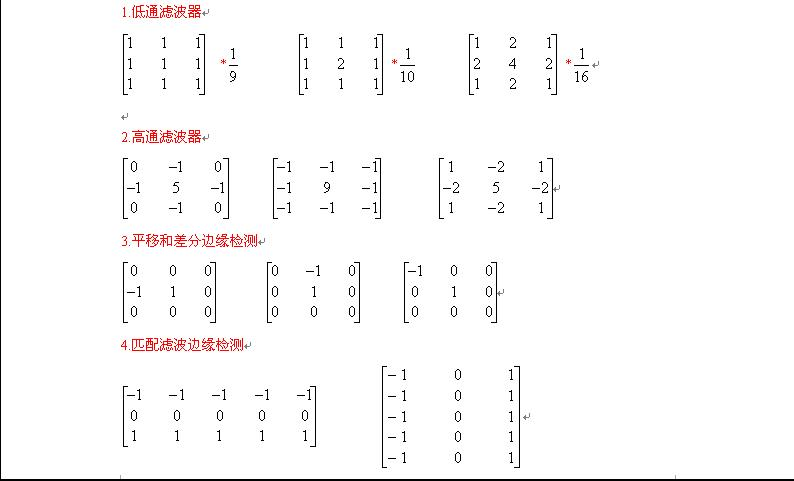2016-06-13 11:20:15 Times_poem 阅读数 8143
• ###### 卷积神经网络与人脸识别实现

本课程讲解人工神经网络的基本知识，而后详细讲解深度学习的经典模型卷积神经网络 CNN，后基于CNN 讲解一个简单的人脸识别系统。知识点包括：神经元、感知机、BP 算法、梯度优化、卷积运算、池化运算、全连接层、激活函数、深度学习python 实现等。

3519 人正在学习 去看看 CSDN讲师

第二部分：图像处理之卷积理解

第三部分：矩阵卷积转换为矩阵相乘

# 第一部分：矩阵的卷积运算详细过程

a=[2 1 3 1;1 2 1 2;2 1 3 2;1 3 1 2];
b=[1 1 1;1 1 1;1 1 1];
c=conv2(a,b,'same');
d=conv2(a,b,'full');
fprintf('\na = \n');
disp(a);
fprintf('\nb = \n');
disp(b);
fprintf('\nc = \n');
disp(c);
fprintf('\nd = \n');
disp(d);

MATALB仿真结果

a =
2     1     3     1
1     2     1     2
2     1     3     2
1     3     1     2

b =
1     1     1
1     1     1
1     1     1

c =
6    10    10     7
9    16    16    12
10    15    17    11
7    11    12     8

d =
2     3     6     5     4     1
3     6    10    10     7     3
5     9    16    16    12     5
4    10    15    17    11     6
3     7    11    12     8     4
1     4     5     6     3     2

（1）    卷积核绕自己的核心元素顺时针旋转180度(这个千万不要忘了)
（2）    移动卷积核的中心元素，使它位于输入图像待处理像素的正上方
（3）    在旋转后的卷积核中，将输入图像的像素值作为权重相乘
（4）    第三步各结果的和做为该输入像素对应的输出像素

a =
2     1     3     1
1     2     1     2
2     1     3     2
1     3     1     2
b =
-1    -1    -1
0     0     0
1     1     1
e =
-1     0     1
-1     0     1
-1     0     1

c =
-3    -4    -5    -3
0     0    -1    -1
-1    -1    -1     0
3     6     6     5

d =
-3    -1     0     4
-4    -2    -1     7
-6    -1     0     5
-4    -1     0     4

# 第二部分：图像处理之卷积理解

f(x) =  - 其中C(k)代表卷积操作数，g(i)代表样本数据, f(x)代表输出结果。1.      读取源图像像素

2.      应用卷积操作数矩阵产生目标图像

3.      对目标图像进行归一化处理

4.      处理边界像素// red color

out3DData[row][col] =in3DData[row][col] +

in3DData[row-1][col] +

in3DData[row+1][col] +

in3DData[row][col-1] +

in3DData[row-1][col-1] +

in3DData[row+1][col-1] +

in3DData[row][col+1] +

in3DData[row-1][col+1] +

in3DData[row+1][col+1];

// green color

out3DData[row][col] =in3DData[row][col] +

in3DData[row-1][col] +

in3DData[row+1][col] +

in3DData[row][col-1] +

in3DData[row-1][col-1] +

in3DData[row+1][col-1] +

in3DData[row][col+1] +

in3DData[row-1][col+1] +

in3DData[row+1][col+1];

// blue color

out3DData[row][col] =in3DData[row][col] +

in3DData[row-1][col] +

in3DData[row+1][col] +

in3DData[row][col-1] +

in3DData[row-1][col-1] +

in3DData[row+1][col-1] +

in3DData[row][col+1] +

in3DData[row-1][col+1] +

in3DData[row+1][col+1];

// find the peak data frominput and output pixel data.

int inpeak = 0;

int outPeak = 0;

for(int row=0; row<srcH; row++) {

for(int col=0; col<srcW; col++) {

if(inpeak < in3DData[row][col]) {

inpeak = in3DData[row][col];

}

if(inpeak < in3DData[row][col]) {

inpeak = in3DData[row][col];

}

if(inpeak < in3DData[row][col]) {

inpeak = in3DData[row][col];

}

if(outPeak < out3DData[row][col]) {

outPeak = out3DData[row][col];

}

if(outPeak < out3DData[row][col]) {

outPeak = out3DData[row][col];

}

if(outPeak < out3DData[row][col]) {

outPeak = out3DData[row][col];

}

}

}

// normalization

double outputScale = ((double) inpeak) / ((double)outPeak);

for(int row=0; row<srcH; row++) {

for(int col=0; col<srcW; col++) {

out3DData[row][col] = (int)(outputScale * out3DData[row][col]);

out3DData[row][col] = (int)(outputScale * out3DData[row][col]);

out3DData[row][col] = (int)(outputScale * out3DData[row][col]);

}

}

# 第三部分：矩阵卷积转换为矩阵相乘

两个矩阵卷积转化为矩阵相乘形式——Matlab应用(这里考虑二维矩阵，在图像中对应)两个图像模糊（边缘）操作，假设矩阵A、B，A代表源图像，B代表卷积模板，那么B的取值决定最后运算的结果。

Matlab中的应用函数——conv2（二维卷积，一维对应conv）

函数给出的公式定义为：同一维数据卷积一样，它的实质在于将卷积模板图像翻转（旋转180），这里等同于一维信号的翻转，然后将卷积模板依次从上到下、从左到右滑动，计算在模板与原始图像交集元素的乘积和，该和就作为卷积以后的数值。

为了验证后续矩阵卷积转化为矩阵相乘，这里给出的conv2的实例描述：

假设矩阵A（4*3）、B（2*3）如下：首先，B需要旋转180，

命令旋转2次90即可：

B = rot90(rot90(B));或者B = rot90(h,2);  结果为：其次：命令conv2函数：

C = conv2(A,B，‘shape’),该函数的具体操作图示：依次计算直至结束，结果数据为：shape的取值有三种，full代表返回卷积以后的全部数据，size为(mA+mB-1,nA+nB-1)的数据；same代表返回卷积以后的原图size (mA,nA)的部分数据；valid返回size为(mA-mB+1,nA-nB+1)的数据，指的是模板元素全部参加运算的结果数据，即源图像和模板的交集为模板。

矩阵卷积转化为矩阵相乘，网上也有很多方法，通俗化表示为：

A×B = B1*A1;

需要针对原始数据与模板数据做变换，变换过程如下：首先进行周期延拓，补零：

M = mA+mB-1 = 5;  N = nA+nB-1 = 5，对应卷积以后full数据大小。

那么初次换换的A和B为：其次对A1和B1分别进行变换

转化B1——针对B1以及转换矩阵方法为：将B1中的每一行向量依次按照B转化为一个方形矩阵Ba~Be，然后针对于每一个方形矩阵按照B矩阵组合成一个新的矩阵B1。B1矩阵的大小为（（mA+mB-1）*（nA+nB-1），（mA+mB-1）*（nA+nB-1））。

转化A1——堆叠向量式

将上个步骤转换的A1按照行向量顺寻依次转化为一个列向量，那么列向量的大小为（（mA+mB-1）*（nA+nB-1），1）大小。针对实例：具体代码为：

周期延拓：

转化A——>A1

1. [m1,n1] = size(A);  [m2,n2] = size(B);
2. m=m1+m2-1;n=n1+n2-1;
5. AA =AA';
6. A1 = AA(:);%%%%

转化B——>B1

1. B2(1,:) = BB(1,:);
2. for i =2:m
3.     B2(i,:) = BB(m-i+2,:);
4. end %%%矩阵a ~ e的重新赋值
5.
6. B4 = zeros(n,n);%%%%%%%每一行转化的方阵
7. B1 = zeros(m*n,m*n);%%%%%最后的矩阵
8. for i =1:m%%%%%%%%几维向量
9.     B = B2(i,:);
10.     if sum(sum(abs(B))==0)
11.         B4 = zeros(n,n);
12.     else
13.        for j = 1:n%%%%%%%元素
14.            for k =0:n-1%%%%%%%%位置(搞定一行向量转化为方阵的形式)
15.                t = mod(j+k,n);
16.                if t==0
17.                   t = n;
18.                end  %%%end if
19.                B4(t,k+1) = B(j);
20.            end %%%end for
21.        end  %%%end for
22.        for  k =0:m-1%%%%%%%%每一个转换矩阵在大矩阵中的位置编号（搞定小方阵在大阵中的位置转化为大方阵的形式）
23.             t = mod(i+k,m);
24.             if t==0
25.                 t = m;
26.             end  %%%end if
27.             B1(k*n+1:(k+1)*n,(t-1)*n+1:t*n) = B4;
28.        end  %%%end for
29.    end %%%end if else
30. end  %%%end for

结果数据转化：

1. Result = B1*A1;
2. Result = reshape(Result,n,m);
3. Result = Result';

得到的结果等同于conv2的数据结果：利用matlab接口更快的实现方法：

还是以5 * 5的原始数据与3*3的卷积核为例子：

代码如下：
1. dd_data = [1,2,4,5,6;6,8,9,1,3;6,8,9,2,4;7,3,5,7,5;1,5,8,9,3]; % 5 *  5
2. f_k = [3,4,5;6,7,8;3,2,1]; % 3 * 3
3. dd_data_f_k = conv2(dd_data,f_k,'full'); % matlab 函数接口
4. dd_data1 = padarray(dd_data,[2 2],'both'); % 扩充原始数据
5. v_dd_data = im2col(dd_data1,[3 3]);% 块数据向量化
6. f_k1 = f_k(:);
7. f_k1 = f_k1(end : -1 :1);
8. f_k1 = f_k1'; % 卷积核的表示
9. dd_data_f_k1 = f_k1 * v_dd_data; % 卷积转化为相乘
10. dd_data_f_k1 = reshape(dd_data_f_k1,[7 7]); % 转化为结果数据

2019-06-25 22:03:56 matt45m 阅读数 276
• ###### 卷积神经网络与人脸识别实现

本课程讲解人工神经网络的基本知识，而后详细讲解深度学习的经典模型卷积神经网络 CNN，后基于CNN 讲解一个简单的人脸识别系统。知识点包括：神经元、感知机、BP 算法、梯度优化、卷积运算、池化运算、全连接层、激活函数、深度学习python 实现等。

3519 人正在学习 去看看 CSDN讲师

## 前言

1.在图像处理中，特别在深度学习图像处理，最常看到图像卷积操作，那什么是卷积呢，卷积就是两个原函数产生一个新的函数，两个函数之间的这种操作就称着卷积，在图像这里的图像卷积也用输入的图像矩阵与一个特定的矩阵进行操作得到一个新的图像。
2.那先了解二维矩阵的卷积是如何运算，之后再讲讲卷积在图像中的应用。

## 卷积运算

1.一个模板矩阵和另一个输入矩阵进行卷积，是使锚点覆盖在待计算矩阵元素上面，然后计算元素值与被覆盖的卷积核中的值的乘积和。将这个和赋值给当前元素，这就是卷积的过程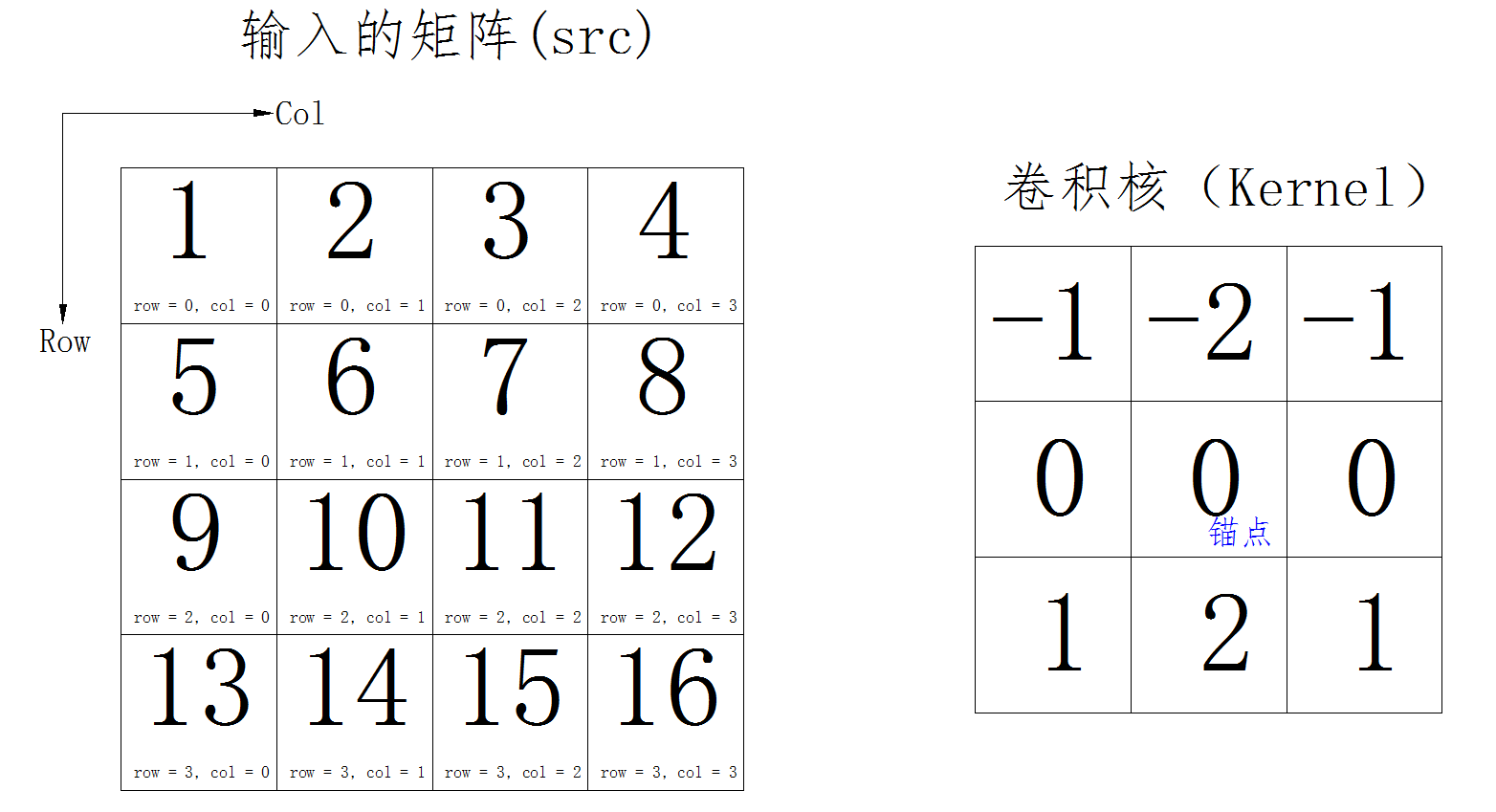2.计算公式如下：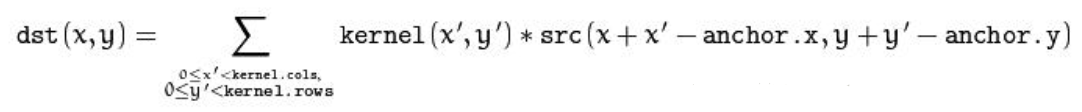3.这里只演示运算方式，先不对卷积核进行180度旋转，也暂时不考虑扩展边界问题，只拿卷积核与输入矩阵做运算，那么一个44的矩阵与一个33的核运算，不超出边界的计算的话，最终只得到一个22的矩阵，步骤如下图。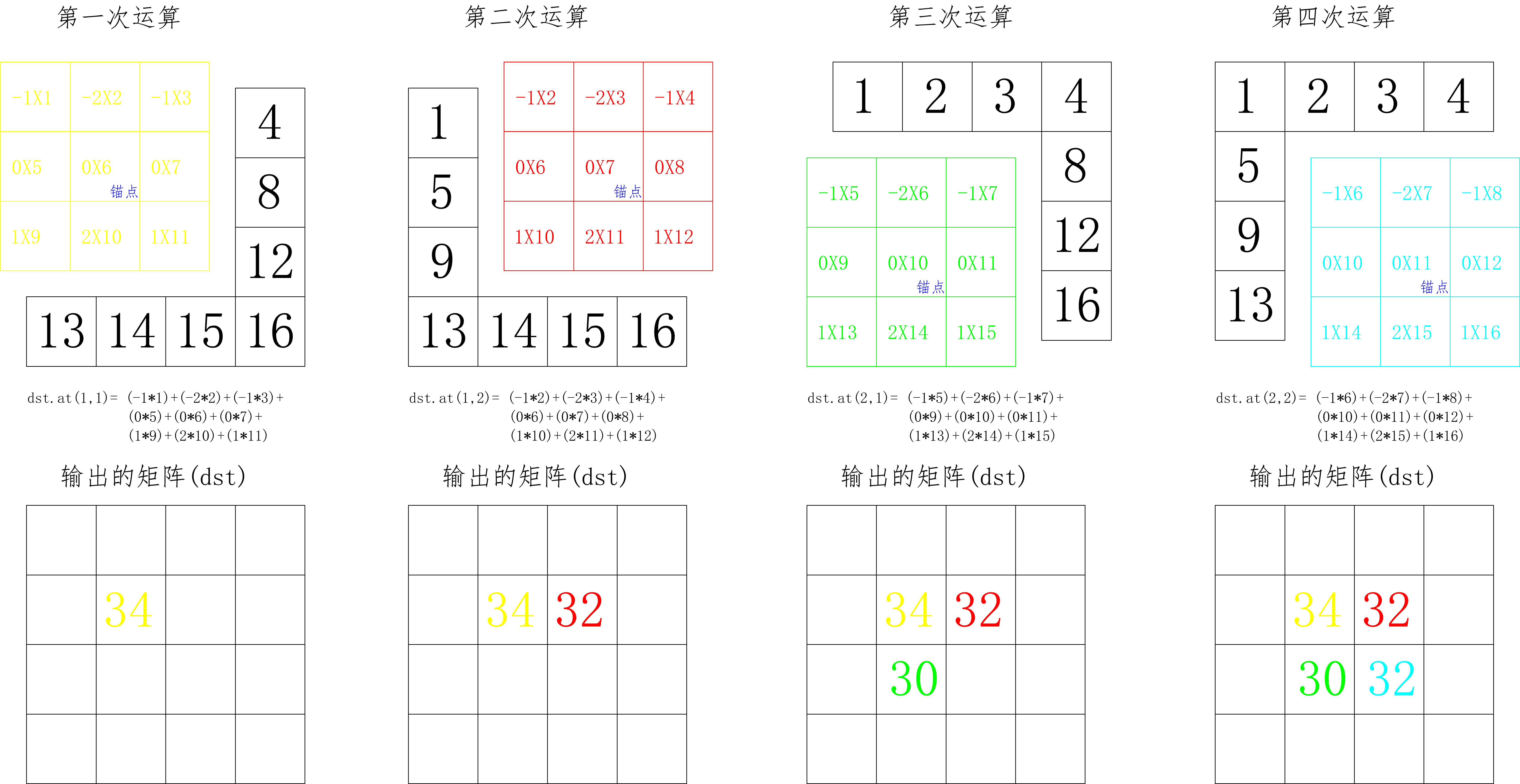2的矩阵，如下图：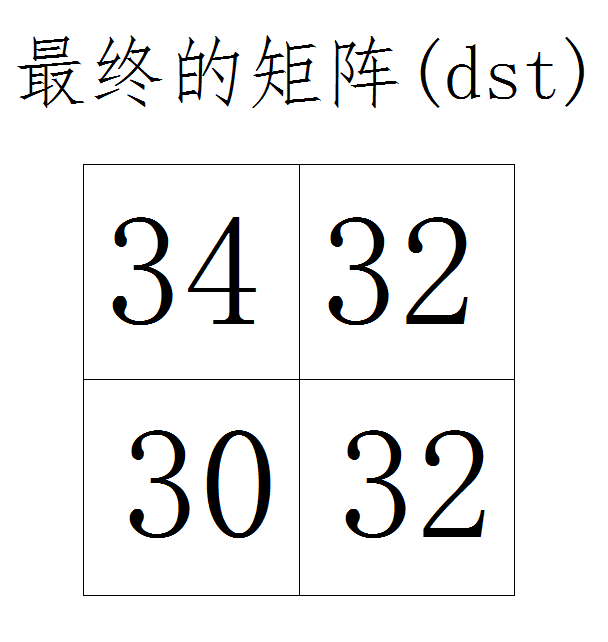4.最终得到的矩阵与原矩阵大小不一致，这肯定不是我们想要的结果，那么这里就要用到扩展边界这个概念，如果不是很清楚什么是边界扩展，可以看我之前的博文，我这里使用特定的值（0）来扩展边界，扩展的大小为卷积核的半径。扩展之后如下图：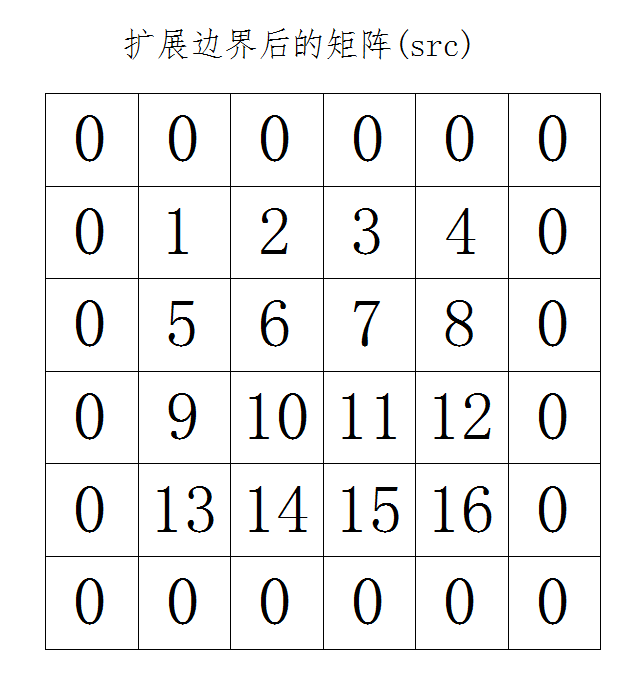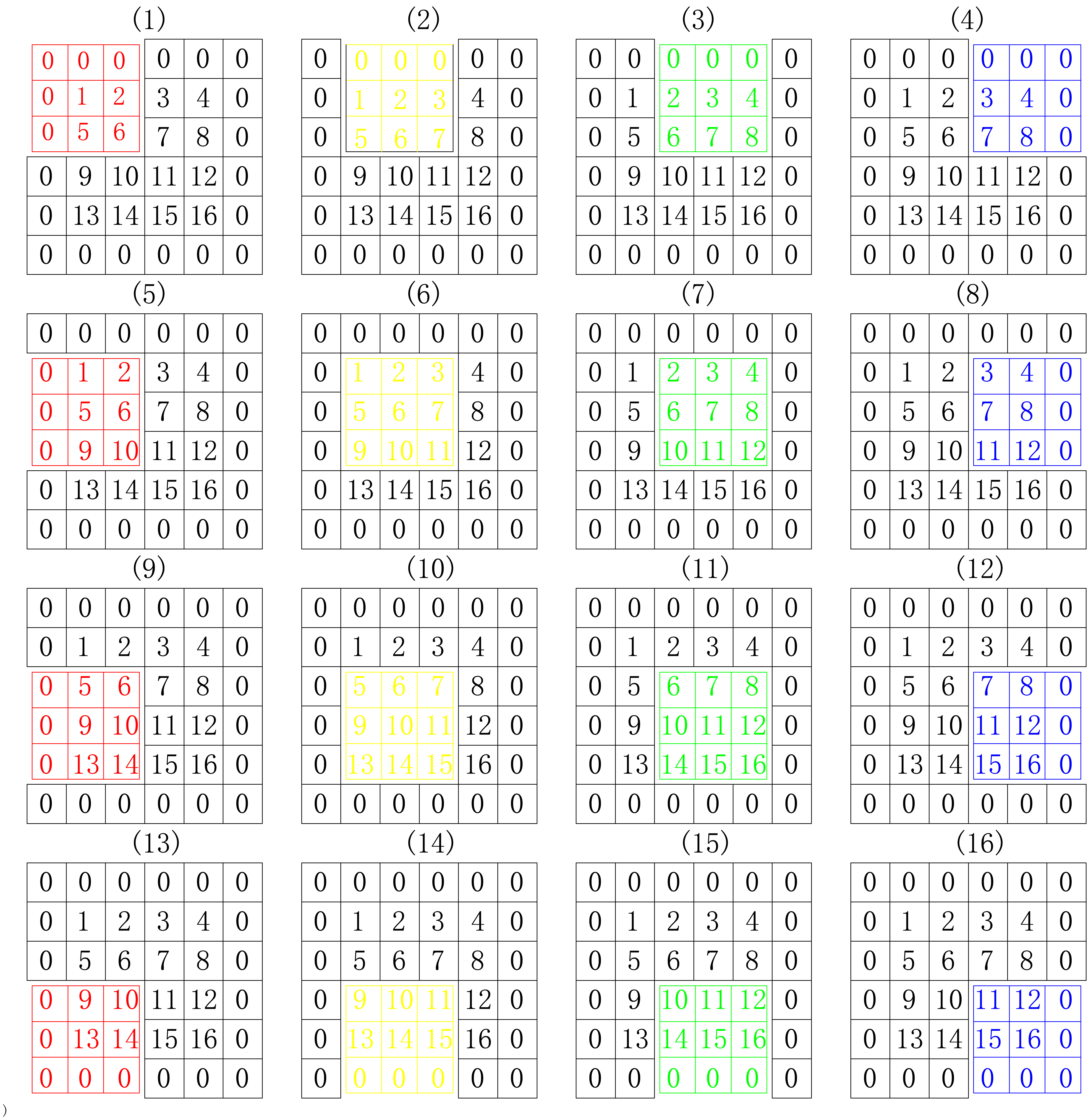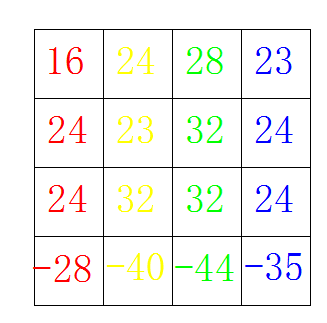2017-01-17 16:49:45 u010830004 阅读数 1660
• ###### 卷积神经网络与人脸识别实现

本课程讲解人工神经网络的基本知识，而后详细讲解深度学习的经典模型卷积神经网络 CNN，后基于CNN 讲解一个简单的人脸识别系统。知识点包括：神经元、感知机、BP 算法、梯度优化、卷积运算、池化运算、全连接层、激活函数、深度学习python 实现等。

3519 人正在学习 去看看 CSDN讲师

第二部分：图像处理之卷积理解

第三部分：矩阵卷积转换为矩阵相乘

# 第一部分：矩阵的卷积运算详细过程

a=[2 1 3 1;1 2 1 2;2 1 3 2;1 3 1 2];
b=[1 1 1;1 1 1;1 1 1];
c=conv2(a,b,'same');
d=conv2(a,b,'full');
fprintf('\na = \n');
disp(a);
fprintf('\nb = \n');
disp(b);
fprintf('\nc = \n');
disp(c);
fprintf('\nd = \n');
disp(d);

MATALB仿真结果

a =
2     1     3     1
1     2     1     2
2     1     3     2
1     3     1     2

b =
1     1     1
1     1     1
1     1     1

c =
6    10    10     7
9    16    16    12
10    15    17    11
7    11    12     8

d =
2     3     6     5     4     1
3     6    10    10     7     3
5     9    16    16    12     5
4    10    15    17    11     6
3     7    11    12     8     4
1     4     5     6     3     2

（1）    卷积核绕自己的核心元素顺时针旋转180度(这个千万不要忘了)
（2）    移动卷积核的中心元素，使它位于输入图像待处理像素的正上方
（3）    在旋转后的卷积核中，将输入图像的像素值作为权重相乘
（4）    第三步各结果的和做为该输入像素对应的输出像素

a =
2     1     3     1
1     2     1     2
2     1     3     2
1     3     1     2
b =
-1    -1    -1
0     0     0
1     1     1
e =
-1     0     1
-1     0     1
-1     0     1

c =
-3    -4    -5    -3
0     0    -1    -1
-1    -1    -1     0
3     6     6     5

d =
-3    -1     0     4
-4    -2    -1     7
-6    -1     0     5
-4    -1     0     4

# 第二部分：图像处理之卷积理解

f(x) =  - 其中C(k)代表卷积操作数，g(i)代表样本数据, f(x)代表输出结果。1.      读取源图像像素

2.      应用卷积操作数矩阵产生目标图像

3.      对目标图像进行归一化处理

4.      处理边界像素// red color

out3DData[row][col] =in3DData[row][col] +

in3DData[row-1][col] +

in3DData[row+1][col] +

in3DData[row][col-1] +

in3DData[row-1][col-1] +

in3DData[row+1][col-1] +

in3DData[row][col+1] +

in3DData[row-1][col+1] +

in3DData[row+1][col+1];

// green color

out3DData[row][col] =in3DData[row][col] +

in3DData[row-1][col] +

in3DData[row+1][col] +

in3DData[row][col-1] +

in3DData[row-1][col-1] +

in3DData[row+1][col-1] +

in3DData[row][col+1] +

in3DData[row-1][col+1] +

in3DData[row+1][col+1];

// blue color

out3DData[row][col] =in3DData[row][col] +

in3DData[row-1][col] +

in3DData[row+1][col] +

in3DData[row][col-1] +

in3DData[row-1][col-1] +

in3DData[row+1][col-1] +

in3DData[row][col+1] +

in3DData[row-1][col+1] +

in3DData[row+1][col+1];

// find the peak data frominput and output pixel data.

int inpeak = 0;

int outPeak = 0;

for(int row=0; row<srcH; row++) {

for(int col=0; col<srcW; col++) {

if(inpeak < in3DData[row][col]) {

inpeak = in3DData[row][col];

}

if(inpeak < in3DData[row][col]) {

inpeak = in3DData[row][col];

}

if(inpeak < in3DData[row][col]) {

inpeak = in3DData[row][col];

}

if(outPeak < out3DData[row][col]) {

outPeak = out3DData[row][col];

}

if(outPeak < out3DData[row][col]) {

outPeak = out3DData[row][col];

}

if(outPeak < out3DData[row][col]) {

outPeak = out3DData[row][col];

}

}

}

// normalization

double outputScale = ((double) inpeak) / ((double)outPeak);

for(int row=0; row<srcH; row++) {

for(int col=0; col<srcW; col++) {

out3DData[row][col] = (int)(outputScale * out3DData[row][col]);

out3DData[row][col] = (int)(outputScale * out3DData[row][col]);

out3DData[row][col] = (int)(outputScale * out3DData[row][col]);

}

}

# 第三部分：矩阵卷积转换为矩阵相乘

两个矩阵卷积转化为矩阵相乘形式——Matlab应用(这里考虑二维矩阵，在图像中对应)两个图像模糊（边缘）操作，假设矩阵A、B，A代表源图像，B代表卷积模板，那么B的取值决定最后运算的结果。

Matlab中的应用函数——conv2（二维卷积，一维对应conv）

函数给出的公式定义为：同一维数据卷积一样，它的实质在于将卷积模板图像翻转（旋转180），这里等同于一维信号的翻转，然后将卷积模板依次从上到下、从左到右滑动，计算在模板与原始图像交集元素的乘积和，该和就作为卷积以后的数值。

为了验证后续矩阵卷积转化为矩阵相乘，这里给出的conv2的实例描述：

假设矩阵A（4*3）、B（2*3）如下：首先，B需要旋转180，

命令旋转2次90即可：

B = rot90(rot90(B));或者B = rot90(h,2);  结果为：其次：命令conv2函数：

C = conv2(A,B，‘shape’),该函数的具体操作图示：依次计算直至结束，结果数据为：shape的取值有三种，full代表返回卷积以后的全部数据，size为(mA+mB-1,nA+nB-1)的数据；same代表返回卷积以后的原图size (mA,nA)的部分数据；valid返回size为(mA-mB+1,nA-nB+1)的数据，指的是模板元素全部参加运算的结果数据，即源图像和模板的交集为模板。

矩阵卷积转化为矩阵相乘，网上也有很多方法，通俗化表示为：

A×B = B1*A1;

需要针对原始数据与模板数据做变换，变换过程如下：首先进行周期延拓，补零：

M = mA+mB-1 = 5;  N = nA+nB-1 = 5，对应卷积以后full数据大小。

那么初次换换的A和B为：其次对A1和B1分别进行变换

转化B1——针对B1以及转换矩阵方法为：将B1中的每一行向量依次按照B转化为一个方形矩阵Ba~Be，然后针对于每一个方形矩阵按照B矩阵组合成一个新的矩阵B1。B1矩阵的大小为（（mA+mB-1）*（nA+nB-1），（mA+mB-1）*（nA+nB-1））。

转化A1——堆叠向量式

将上个步骤转换的A1按照行向量顺寻依次转化为一个列向量，那么列向量的大小为（（mA+mB-1）*（nA+nB-1），1）大小。针对实例：具体代码为：

周期延拓：

转化A——>A1

1. [m1,n1] = size(A);  [m2,n2] = size(B);
2. m=m1+m2-1;n=n1+n2-1;
5. AA =AA';
6. A1 = AA(:);%%%%

转化B——>B1

1. B2(1,:) = BB(1,:);
2. for i =2:m
3.     B2(i,:) = BB(m-i+2,:);
4. end %%%矩阵a ~ e的重新赋值
5.
6. B4 = zeros(n,n);%%%%%%%每一行转化的方阵
7. B1 = zeros(m*n,m*n);%%%%%最后的矩阵
8. for i =1:m%%%%%%%%几维向量
9.     B = B2(i,:);
10.     if sum(sum(abs(B))==0)
11.         B4 = zeros(n,n);
12.     else
13.        for j = 1:n%%%%%%%元素
14.            for k =0:n-1%%%%%%%%位置(搞定一行向量转化为方阵的形式)
15.                t = mod(j+k,n);
16.                if t==0
17.                   t = n;
18.                end  %%%end if
19.                B4(t,k+1) = B(j);
20.            end %%%end for
21.        end  %%%end for
22.        for  k =0:m-1%%%%%%%%每一个转换矩阵在大矩阵中的位置编号（搞定小方阵在大阵中的位置转化为大方阵的形式）
23.             t = mod(i+k,m);
24.             if t==0
25.                 t = m;
26.             end  %%%end if
27.             B1(k*n+1:(k+1)*n,(t-1)*n+1:t*n) = B4;
28.        end  %%%end for
29.    end %%%end if else
30. end  %%%end for

结果数据转化：

1. Result = B1*A1;
2. Result = reshape(Result,n,m);
3. Result = Result';

得到的结果等同于conv2的数据结果：利用matlab接口更快的实现方法：

还是以5 * 5的原始数据与3*3的卷积核为例子：

代码如下：
1. dd_data = [1,2,4,5,6;6,8,9,1,3;6,8,9,2,4;7,3,5,7,5;1,5,8,9,3]; % 5 *  5
2. f_k = [3,4,5;6,7,8;3,2,1]; % 3 * 3
3. dd_data_f_k = conv2(dd_data,f_k,'full'); % matlab 函数接口
4. dd_data1 = padarray(dd_data,[2 2],'both'); % 扩充原始数据
5. v_dd_data = im2col(dd_data1,[3 3]);% 块数据向量化
6. f_k1 = f_k(:);
7. f_k1 = f_k1(end : -1 :1);
8. f_k1 = f_k1'; % 卷积核的表示
9. dd_data_f_k1 = f_k1 * v_dd_data; % 卷积转化为相乘
10. dd_data_f_k1 = reshape(dd_data_f_k1,[7 7]); % 转化为结果数据